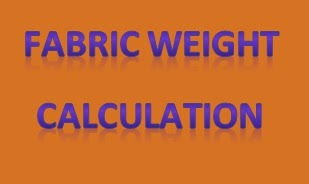# Textile Calculation

Problems and solutions of textile

## Fabric Weight Calculation

How to Calculate Fabric Weight
S. M. Hossen Uzzal
Production Officer
Monno Fabrics Ltd., Manikgonj
Email: uhossen@gmail.com

Fabric Weight:
Fabric weight is a most important characteristic of a fabric. It is one of the ways in which fabrics are classified. Fabric weights are measured in a few different ways. Generally, the fabric weight is measured by weighing a standardized width of a yard or meter on a scale. Fabric weights are also occasionally determined by weighing square yards of a quality, or by weighing yards per one pound. The weight of the one-yard or meter is recorded in ounces or grams and the fabric is then classified by its weight. The weight of textiles is measured in ounces in the U.S. and in Asia, and is measured in grams in Europe.Fabric Weight Calculation:

EPI X PPI
Fabric specification = ……………………………… X Fabric width
Warp count X Weft count

Or,

Warp count X Weft count
Fabric specification = ………………………………….. X Fabric width
EPI X PPI

Problem:

10 X 8
Fabric specification = …………. X 60”
. Calculate the fabric weight of 1000m.
70 X 50

Solution:

EPI = 70 and warp count = 10

Total no of warp = 70 X 60 = 4200

Length of each end = 1000m + 1000 X 3%            [3% crimp]
= 1030m

Total length of warp = 4200 X 1030 = 4326000m

Now, we know

L X w
Ne = ………..
W X l

4200 X 1030 X 0.4536
Or, 10 = ……………………………………
W X 840 X 0.91

4200 X 1030 X 0.4536
Or, W = …………………………
10 X 840 X 0.91

Or, W = 256.71 kg

PPI = 50 and weft count = 8

Total no of weft = 50 X 39.37 X 1000

Length of each weft = 60 + 60 X 5%             [Crimp 5%]
= 63”
= 1.6m

Total length of weft = 50 X 39.37 X 1000 X 1.6 m

Again,

L X w
Ne = ………..
W X l

50 X 39.37 X 1000 X 1.6
Or, 8 = ……………………………
W X 840 X 0.91

50 X 39.37 X 1000 X 1.6
Or, W = …………………………….
8 X 840 X 0.91

Or, W = 233.62 kg

Total fabric weight = 256.71 + 233.62 kg

= 490.33 kg (ANS)